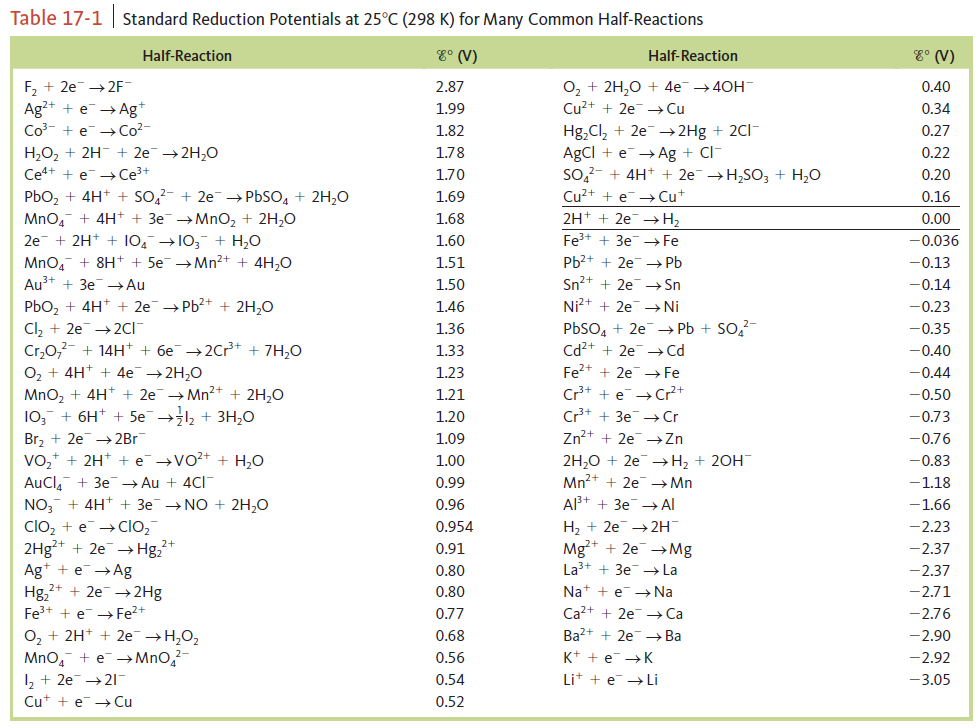# Problem: Exercise 46. Give the balanced cell equation and determine E° for the galvanic cells based on the following half-reactions. Standard reduction potentials are found in Table 17‑1.a. Cr2O72- + 14H+ + 6e- → 2Cr 3+ + 7H2O    H2O2 + 2H+ + 2e- → 2H2Ob. 2H + + 2e- → H2    Al 3+ + 3e- → AlCalculate the maximum amount of work that can be obtained from the galvanic cells at standard conditions in Exercise 46.

###### FREE Expert Solution

Recall:

Lose               Gain
Electron         Electrons
Oxidation       Reduction

cathode → reduction → oxidation number decreases

anode → oxidation → oxidation number increases

For ions: charge of the ion = oxidation number
For neutral atoms/compound: oxidation number = 0

A.

Cr2O72- + 14H+ + 6e- → 2Cr 3+ + 7H2O

[H2O2 + 2H+ + 2e- → 2H2O]×3

98% (366 ratings)###### Problem Details

Exercise 46. Give the balanced cell equation and determine E° for the galvanic cells based on the following half-reactions. Standard reduction potentials are found in Table 17‑1.

a. Cr2O72- + 14H+ + 6e- → 2Cr 3+ + 7H2O
H2O2 + 2H+ + 2e- → 2H2O

b. 2H + + 2e- → H2
Al 3+ + 3e- → AlCalculate the maximum amount of work that can be obtained from the galvanic cells at standard conditions in Exercise 46.

Frequently Asked Questions

What scientific concept do you need to know in order to solve this problem?

Our tutors have indicated that to solve this problem you will need to apply the Cell Potential concept. If you need more Cell Potential practice, you can also practice Cell Potential practice problems.

What professor is this problem relevant for?

Based on our data, we think this problem is relevant for Professor Egolf's class at Penn State University Park.

What textbook is this problem found in?

Our data indicates that this problem or a close variation was asked in Chemistry: An Atoms First Approach - Zumdahl Atoms 1st 2nd Edition. You can also practice Chemistry: An Atoms First Approach - Zumdahl Atoms 1st 2nd Edition practice problems.